# Excel 两个工作表 多条件查询重复数据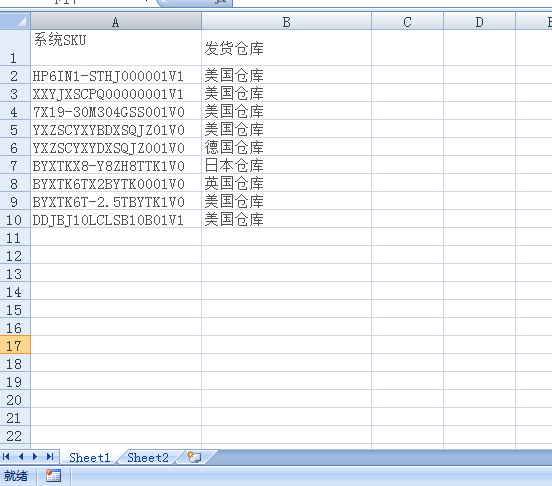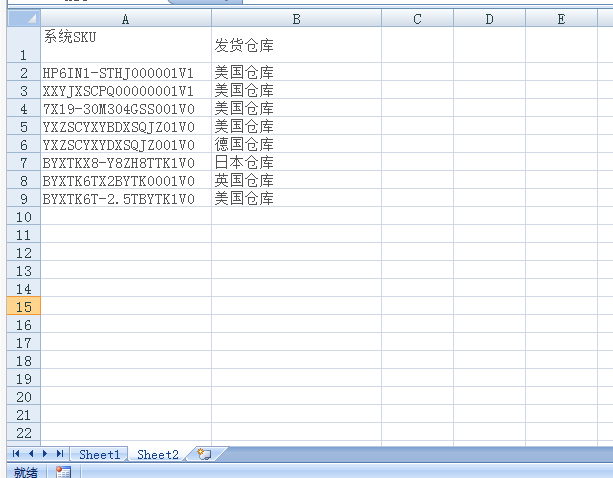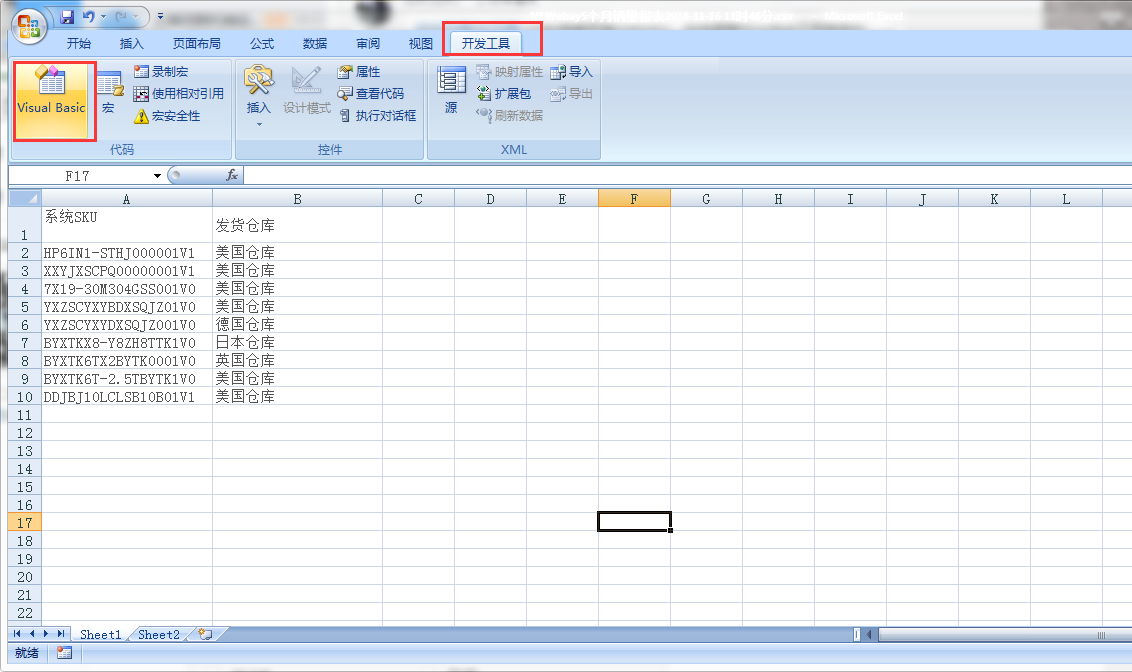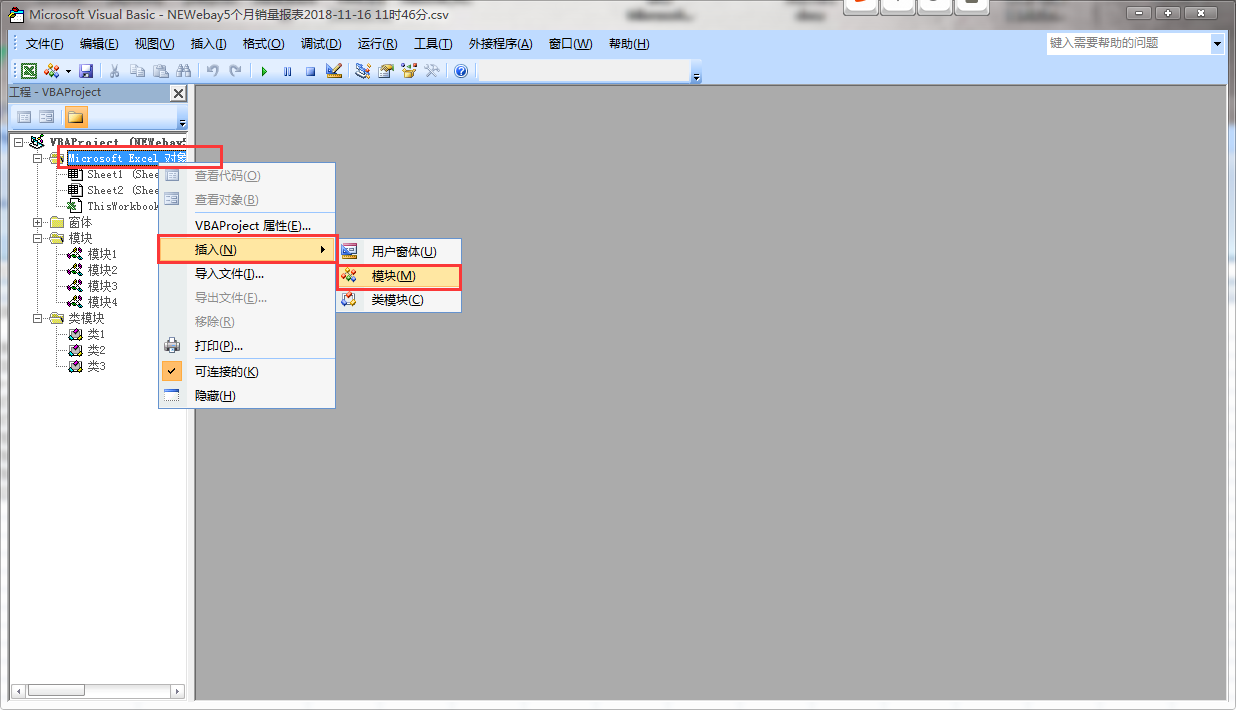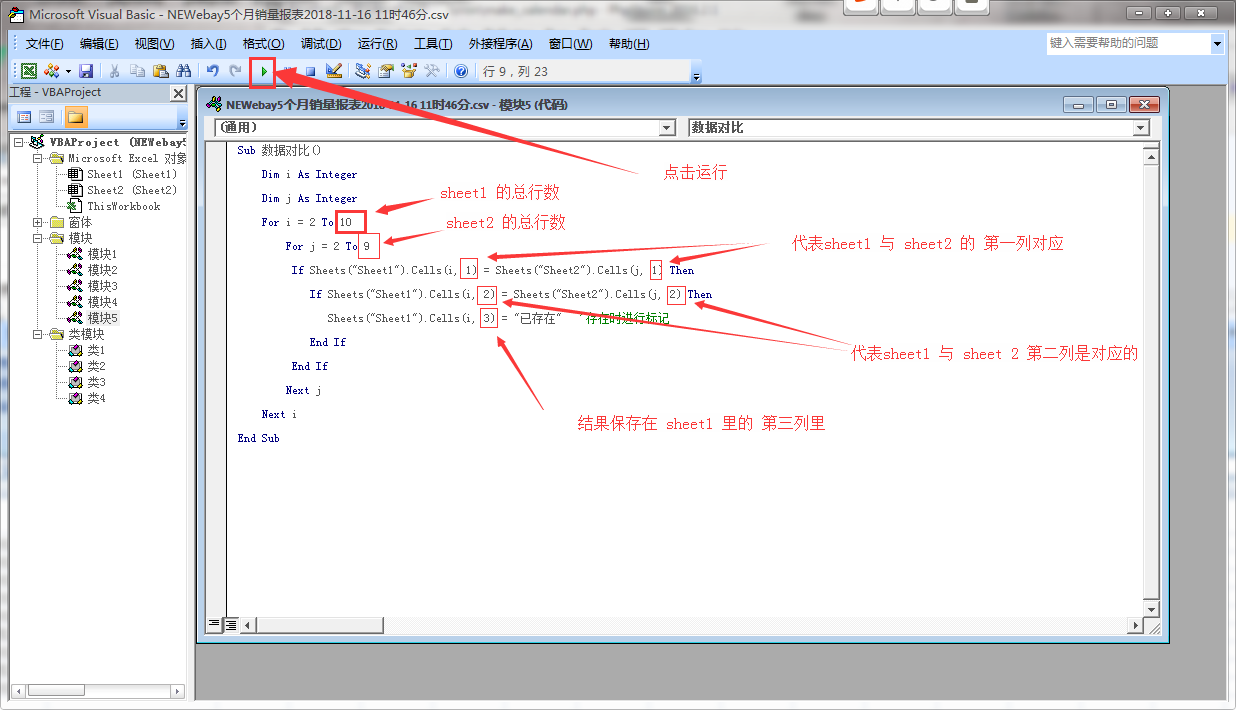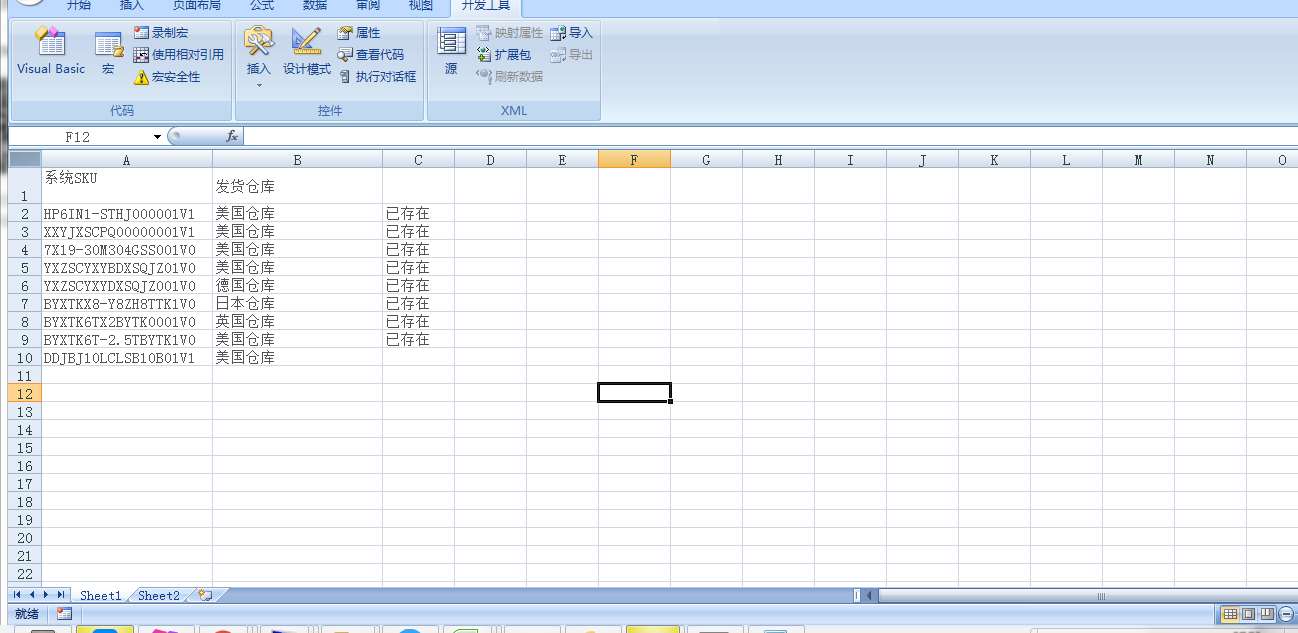``````Sub 数据对比()

Dim i As Integer

Dim j As Integer

For i = 2 To 10

For j = 2 To 9

If Sheets("Sheet1").Cells(i, 1) = Sheets("Sheet2").Cells(j, 1) Then

If Sheets("Sheet1").Cells(i, 2) = Sheets("Sheet2").Cells(j, 2) Then

Sheets("Sheet1").Cells(i, 3) = "已存在"   '存在时进行标记

End If

End If

Next j

Next i

End Sub

``````